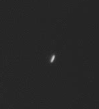# Problems of the Week

Contribute a problem

# 2017-05-29 Basic

A person is driving past a clock tower. The clock seen in the car's side-view mirror is this:What is the actual time?We use a light microscope to see tiny objects invisible to the naked eye. A light microscope works by magnifying light waves with high-powered lenses. Some of the more powerful light microscopes can magnify an object thousands of times. The E. coli cells shown in the clip above are each so small that thousands of them could fit across the diameter of a needle.

Is it true that if we keep on zooming in on a specimen using more and more powerful lenses, then we can even see the atoms of the specimen?

How many of the following numbers are divisible by 3?

$\begin{array}{l} 1 \\ 12 \\ 123 \\ 1234 \\ 12345 \\ 123456 \\ 1234567 \\ 12345678 \\ 123456789 \\ \end{array}$How many triangles of any size are there in the figure above?

$\dfrac{n+1}{1},\ \dfrac{n+2}{2},\ \dfrac{n+3}{3},\ \dfrac{n+4}{4},\ \dfrac{n+5}{5}$

With $n=0$, all of the above numbers are integers (they all equal 1). What is the next positive integer $n$ such that all of the above numbers are integers?

×